NEET  >  Test: Molecular Velocity

# Test: Molecular Velocity - NEET

Test Description

## 20 Questions MCQ Test Chemistry Class 11 - Test: Molecular Velocity

Test: Molecular Velocity for NEET 2023 is part of Chemistry Class 11 preparation. The Test: Molecular Velocity questions and answers have been prepared according to the NEET exam syllabus.The Test: Molecular Velocity MCQs are made for NEET 2023 Exam. Find important definitions, questions, notes, meanings, examples, exercises, MCQs and online tests for Test: Molecular Velocity below.
Solutions of Test: Molecular Velocity questions in English are available as part of our Chemistry Class 11 for NEET & Test: Molecular Velocity solutions in Hindi for Chemistry Class 11 course. Download more important topics, notes, lectures and mock test series for NEET Exam by signing up for free. Attempt Test: Molecular Velocity | 20 questions in 20 minutes | Mock test for NEET preparation | Free important questions MCQ to study Chemistry Class 11 for NEET Exam | Download free PDF with solutions
 1 Crore+ students have signed up on EduRev. Have you?
Test: Molecular Velocity - Question 1

### Direction (Q. Nos. 1-15) This section contains 15 multiple choice questions. Each question has four choices (a), (b), (c) and (d), out of which ONLY ONE option is correct. Q. Pressure and temperature needed to confine 1.0 x 1025 gas molecules, each with a mass of 1.0x 1025 kg and root mean square velocity of 1.0x 103ms-1 in a 1.0 m3 container are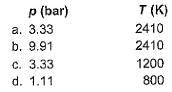Detailed Solution for Test: Molecular Velocity - Question 1

Applying the formula
PV = 1/3(mNV2rms)
Where m = mass of each particle and N = no of particles
We get P = 3.33*105 pascal or 3.3 bar
Applying the formula
Vrms = √(3RT/M)
We get T = 2405 K(approx)
So option a is correct.

Test: Molecular Velocity - Question 2

### At 298 K, which of the following gases has the lowest average molecular speed?

Detailed Solution for Test: Molecular Velocity - Question 2

Under the same conditions the average kinetic energy of the gases should be equal.
speed of gas1/speed of gas 2 = square root of (molar mass of gas2/molar mass of gas 1)
So gases with small molar mass will move quicker
eg speed of N2/speed of CO2 = sq. rt (44/28) = 1.25
speed of N2/speed of F2 = sq rt. (38/28) = 1.16
So order is N2, F2 and CO2
then we concluded right ans. is (A)

Test: Molecular Velocity - Question 3

### By what ratio will the average velocity of the molecules in a gas change when the temperature is raised from 50°C to 200°C?

Detailed Solution for Test: Molecular Velocity - Question 3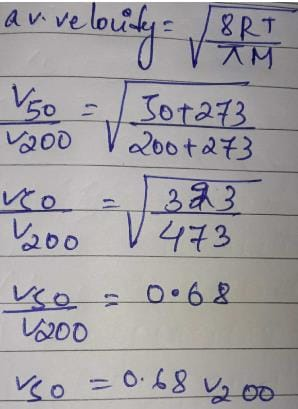This is the correct answer. If we take v200/v50, then we will get option b. But that is not according to the question.

Test: Molecular Velocity - Question 4

The root mean square velocity of a monoatomic gas (molar mass = m g mol-1) is u. Its kinetic energy per mole (E) is related to u by equation

Detailed Solution for Test: Molecular Velocity - Question 4

K.E = ½ × mass × Vrms2
Dividing both sides by moles
K.E/Moles = ½ × mass × Vrms2 /Mole
K.E/mole = ½ Vrms2 × molar mass
Given, K.E/mole = E, molar mass = m and Vrms = u
E = ½ × m × u2
u2 = 2E/m
u = √(2E/m)

Test: Molecular Velocity - Question 5

Root mean square velocity (u) is dependent on temperature. Value of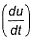is

Detailed Solution for Test: Molecular Velocity - Question 5

The correct answer is option B
Root mean square is v=under root 3RT/M
so we simply do differentiation with respect to temperature DU/DT=D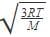/DT
So,
=  3R/2M

Test: Molecular Velocity - Question 6

The pressure needed to confine 1.00 mole of N2 molecules to 24.8 L flask is 1.0 bar. Thus, root mean square velocity of the gas assuming ideal behaviour is

Detailed Solution for Test: Molecular Velocity - Question 6

Vrms = √ 3RT/M = √ 3pV/M
= √ (3×105 × 24.8×10-3)/28
= 51.4 ms-1
So according to me, option A is correct

Test: Molecular Velocity - Question 7

The ratio between the root mean square speed of H2 gas at 50 K and that of O2 gas at 800 K is

[IIT JEEE 1996]

Detailed Solution for Test: Molecular Velocity - Question 7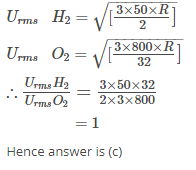Test: Molecular Velocity - Question 8

The rms velocity of hydrogen gas is √7 times of the rms velocity of nitrogen gas. If T is the temperature of the gas, then

[IIT JEE 2000]

Detailed Solution for Test: Molecular Velocity - Question 8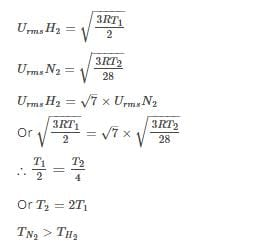Test: Molecular Velocity - Question 9

For CO2, given that average velocity at T, is equal to most probable velocity at T2.

Thus, T1/T2 is

Test: Molecular Velocity - Question 10

For gaseous state, if most probable speed is denoted by C*, average speed by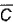and mean square speed by C, then for a large number of molecules, the ratios of these speeds are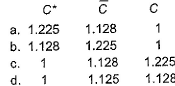[JEE Main 2013]

Detailed Solution for Test: Molecular Velocity - Question 10

RMS velocity = √ (3RT/M) = 1.732k
Average velocity = √ (8RT/πM)
Most probable velocity = √ (2RT/M)
=√2:√8/π:√3=1:1.128:1.225

So, C∗:C:C=1:1.128:1.225

Test: Molecular Velocity - Question 11

Molar mass of a certain gas B is double that of A.

Also RMS velocity of gas A is 200 ms -1 at a certain temperature. RMS velocity of gas B at the temperature half that of A is

Detailed Solution for Test: Molecular Velocity - Question 11

The correct answer is Option B.
Molar mass of B  is double that of A.
2 MA = 8 MB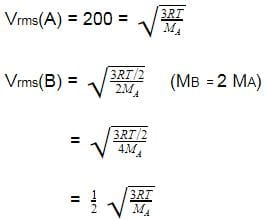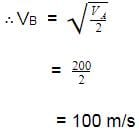Test: Molecular Velocity - Question 12

A vessei of capacity 1 dm3 contains 1.03 x 1023 H2 molecules exerting a pressure of 101.325 kPa. Calculate RMS speed and average speed.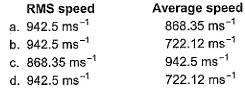Detailed Solution for Test: Molecular Velocity - Question 12

V = 1dm3 = 10-3m3
M.w = 2*10-3g
P = 101.325kPa
= 1.03*1023 H2 mol
No of moles = number of molecules/NA
= 1.03/6.023
= 101.325 * 103 * 10-3 = 8.314 * 1.03/6.023 * T
T = 71.27K
uavg = (8RT/πM)1/2
= [(8*8.314*71.27)/(3.142*2*10-3)]^1/2
= 868.53 m/s
urms = (3RT/M)1/2
[(3*8.314*71.27)/(2*10-3)]1/2
= 942.5 m/s

Test: Molecular Velocity - Question 13

The molecular velocity of any gas is

[AlEEE 2011]

Detailed Solution for Test: Molecular Velocity - Question 13

The molecular velocity of any gas whether it is average velocity, root mean square velocity and most probable velocity. It must be proportional to the square to the square root of absolute temperature.

Test: Molecular Velocity - Question 14

Distribution of molecules with velocity is represented by the curve as shown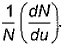Select the correct statement(s).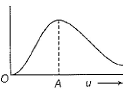*Multiple options can be correct
Test: Molecular Velocity - Question 15

Select the correct statement (s).

*Answer can only contain numeric values
Test: Molecular Velocity - Question 16

Direction (Q. Nos. 16 and 17) This section contains 2 questions. when worked out will result in an integer from 0 to 9 (both inclusive).

Q. Temperature of an ideal gas is changed from -173°C to 127°C. Thus average velocity changes by .......... times.

Detailed Solution for Test: Molecular Velocity - Question 16

T1 = 273 - 173 = 100K
T2 = 273 + 127 = 400K
c1/c2 = (T1/T2)^1/2
c1/c2 = (100/400)^1/2
c1/c2 = (1/4)^1/2
c1/c2 = 1/2
c2 = 2c1

*Answer can only contain numeric values
Test: Molecular Velocity - Question 17

At what temperature (in °C) root mean square velocity of O2 gas at 300 K is equal to most probable velocity of Ne(20 g mol-1)?

Detailed Solution for Test: Molecular Velocity - Question 17

Vrms = √(3RT/M)
Vmp 0= √(2RT/M)
Putting corresponding values,
3×300/32 = 2×T/20
On solving, we get T = 281.5K
T in °C = 281.5-273 = 8.1 °C = 8

Test: Molecular Velocity - Question 18

Direction (Q. Nos. 18-20) This section contains a paragraph, wach describing theory, experiments, data etc. three Questions related to paragraph have been given.Each question have only one correct answer among the four given options (a),(b),(c),(d).

Distribution of molecular velocities was studied by Maxwell and is given by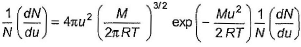is also called probability P.

Q.
Based on following curves,
arrange temperatures T1 , T2 and T3 in increasing order,​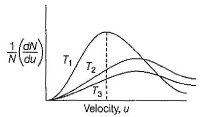Detailed Solution for Test: Molecular Velocity - Question 18

The correct answer is option (C)
The correct order of temperature is,
T1  < T2 < T3
For a particular speed, the number of molecules having that speed is more at lower temperature than the number of molecules having that speed is more at higher temperature.

Test: Molecular Velocity - Question 19

Distribution of molecular velocities was studied by Maxwell and is given byis also called probability P.

Q. Following is the distribution of molecules at 300 K for gases X, Y and Z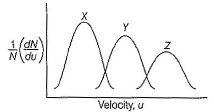Thus,

Test: Molecular Velocity - Question 20

Distribution of molecular velocities was studied by Maxwell and is given byis also called probability P.

Q.
As velocity increases, probability also increases. It is maximum at a particular velocity. This is called most probable velocity. At this point, variation of probability P with velocity u is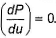Thus, based on this, velocity is

Detailed Solution for Test: Molecular Velocity - Question 20

The correct answer is Option B.
The speed of an individual gas particle is: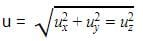The most probable speed is the maximum value on the distribution plot.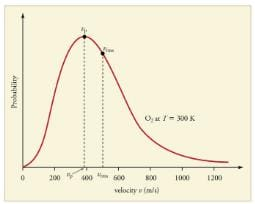This is established by finding the velocity when the derivative of Equation;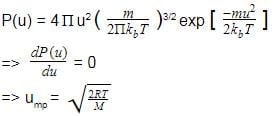## Chemistry Class 11

195 videos|336 docs|190 tests
Information about Test: Molecular Velocity Page
In this test you can find the Exam questions for Test: Molecular Velocity solved & explained in the simplest way possible. Besides giving Questions and answers for Test: Molecular Velocity, EduRev gives you an ample number of Online tests for practice

## Chemistry Class 11

195 videos|336 docs|190 tests

### How to Prepare for NEET

Read our guide to prepare for NEET which is created by Toppers & the best Teachers